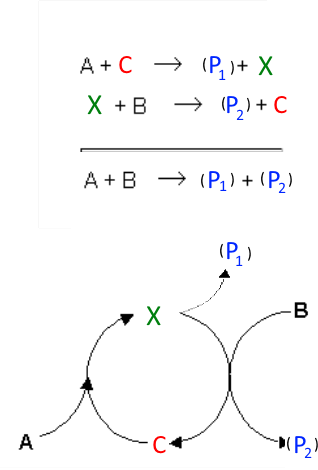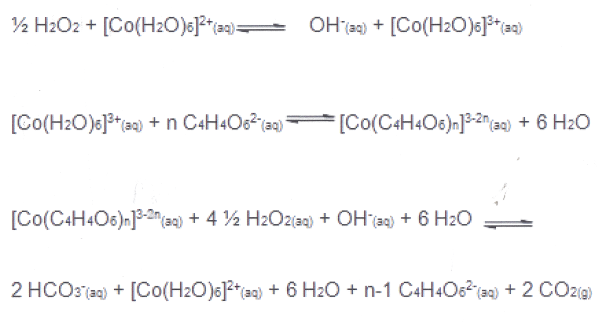Search:

# The homogeneous catalysis

## Definition

Homogeneous catalysis is when the catalyst is in the same phase as the reactants and products.

## Frequent caseOften one of the reactants (here $A$) together with the catalyst (here $C$) produce optionally a product (here $P_1$ ) and an intermediate (here $X$), which in turn reacts with the second reactant (here $B$) to optionally form a product (here $P_2$) and restitutes the catalyst. Example 1: Propagation step in the →       radicalar substitution of alkanes: $Br\cdot$ $+$ $CH_4$ $\rightarrow$ $CH_3\cdot$ $+$ $HBr$ $CH_3\cdot$ $+$ $Br_2$ $\rightarrow$ $CH_3Br$ $+$ $Br\cdot$ with: $A$ $=$ $CH_4$ $C$ $=$ $Br\cdot$ $B$ $=$ $Br_2$ $P_1$ $=$ $HBr$ $P_2$ $=$ $CH_3Br$ Example 2: Reaction of iodide and persulfate in the presence of some $Fe^{3+}$:

$S_2O_8^{2-}$ $+$ $2Fe^{2+}$ $\longrightarrow$ $2SO_4^{2-}$ $+$ $2Fe^{3+}$ $2Fe^{3+}$ $+$ $2I^-$ $\longrightarrow$ $2Fe^{2+}$ $+$ $I_2$ with: $A$ $=$ $S_2O_8^{2-}$ $C$ $=$ $Fe^{2+}$ $B$ $=$ $I^-$ $P_1$ $=$ $SO_4^{2-}$ $P_2$ $=$ $I_2$

## Variant

Many variants are possible, for example: In the case of →   the reaction of hydrogen peroxide and potassium sodium tartrate things are getting more complicated:1 Oxidation of catalyst $Co^{2+}$ (rose) to $Co^{3+}$ 2 Formation of a the complex ion cobalt(III)tartrate (green) 3 Redox reaction: Tartrate and hydrogen peroxide are oxidised to $H_2O$ and $CO_2$ $Co^{3+}$ is reduced to $Co^{2+}$ (rose) and therefore restituted!

Detailed reactions are